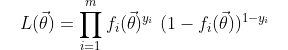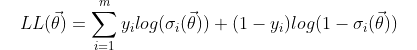top of pageSearch

# Logistic Regression & Cross-Entropy

Updated: Nov 7

Logistic regression is a very popular machine learning algorithm that is primarily used for binary classification tasks. It is a model that applies the logistic (or sigmoid) function to a linear combination of input features to produce a probability estimate for class membership. With its simplicity and interpretability, logistic regression has found widespread applications in various domains, including healthcare, marketing, finance, and social sciences. In this article, we will explore logistic regression in detail, discussing important concepts such as the sigmoid function, log likelihood and cross-entropy.

The sigmoid function, also known as the logistic function, plays a fundamental role in logistic regression. It squashes the output of a linear combination into the range [0, 1], representing a valid probability estimate. The sigmoid function is defined as:Note that the sigmoid is a monotonically increasing function from 0 to 1 and has two horizontal asymptotes at y = 0 and y = 1. This means that the slope of the sigmoid is very small as x approaches -infinity and +infinity while its maximum in the middle at z = 0. Let's take a bit of time to plot the sigmoid using Python and see what it looks like.

```import matplotlib.pyplot as plt
import numpy as np

def sigmoid(x):
return 1/(1 + np.exp(-x))

x = np.linspace(-10, 10, 100000)
y = sigmoid(x)

plt.plot(x, y)
plt.xticks(ticks=np.arange(-10, 10, 1))
plt.yticks(ticks=np.arange(0, 1, 0.1))
plt.grid()
plt.xlabel("z")
plt.ylabel("sigma(z)")
plt.title("SIGMOID FUNCTION")
plt.show()```Please note the following very elegant result regarding the sigmoid derivativeLet's now consider a dataset X of size mxn, i.e. m data points and n features, and an nx1 vector of parameters theta.Please note that a bias vector of ones can easily be added as the first column of X without changing the result of the following mathematical considerations. Let's represent the labels of this dataset as a vector Y, i.e.Our objective is to learn the parameters vector theta that maximises the data likelihoodLet's now define the probability density function which will be estimate of our model to be the sigmoid functionLet's now consider the Log Likelihood as it will be much easier to work withWe can now define the Binary Cross-Entropy Cost function asNote that that the division by m makes the cost function more interesting as it provides the average cost per data point. On the other hand, the minus sign helps us turn the log likelihood maximisation problem into a minimisation problem where we can use gradient descent.Let's now calculate the derivative of the cost function with respecter to the individual parameter theta_j which can be any of the parameters of the parameter vector theta.We can now write the gradient descent update in compact matrix formwhere alpha is the learning rate.

### Generalising the Cross-Entropy

Please note that there exist a generalisation of the sigmoid and cross-entropy for classification problems that involve more than 2 classes which are often used for neural networks and deep learning. More specifically, the sigmoid function can be generalised using the softmax functionwhere K is the number of classes. Please note that the softmax function sums up to one over all classes as expected, given that it outputs k probabilities for each of the k outputs.

The cross-entropy loss function gets generalised towhere g(z) is any non-linear transformation applied to the input data point. Please feel free to check that the cross-entropy for K classes reduces to the binary cross-entropy when K = 2.

Finally, the cost function becomes### Applications of Logistic Regression

Logistic regression finds applications in various domains where binary classification is required. Some notable applications include:

Healthcare: Logistic regression is used for disease prediction, risk assessment, and early diagnosis. For example, it can be used to predict the likelihood of a patient having a particular disease based on their symptoms, medical history, and genetic information.

Marketing: Logistic regression is widely used in customer churn prediction, customer segmentation, and response modeling. It helps businesses understand and target specific customer groups more effectively, enabling personalized marketing strategies.

Finance: Logistic regression is employed in credit scoring models to assess the creditworthiness of individuals based on their financial behavior, demographic information, and credit history. It aids in making accurate credit decisions and managing the risk associated with lending.

Social Sciences: Logistic regression is used in social sciences research to study various phenomena, such as voting behavior, consumer preferences, and opinion mining. It helps analyze categorical outcomes and identify the factors that influence them.

### Conclusion

Logistic regression is a powerful and widely used algorithm that facilitates binary classification tasks. By applying the sigmoid function, it transforms a linear prediction into a probability estimate while maintaining interpretability. With its broad applications and ease of implementation, logistic regression continues to be a valuable tool in the field of machine learning.

The log likelihood/cross-entropy loss, which is also widely used in Deep Learning classification tasks, helps us to run gradient descent efficiently during training and has a mathematically very elegant weights update formula.